Next: Motion with constant acceleration Up: Motion in 3 dimensions Previous: Vector velocity and vector

## Motion with constant velocity

An object moving in 3 dimensions with constant velocitypossesses a vector displacement of the form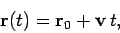(61)

where the constant vector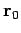is the displacement at time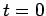. Note that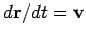and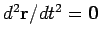, as expected. As illustrated in Fig. 14, the object's trajectory is a straight-line which passes through pointat timeand runs parallel to vector.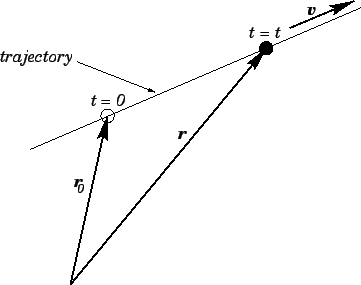Richard Fitzpatrick 2006-02-02